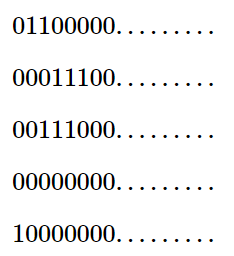[Solution] Ezzat and Grid Solution Codeforces

[Solution] Ezzat and Grid Solution Codeforces

Moamen was drawing a grid of nn rows and 109109 columns containing only digits 00 and 11. Ezzat noticed what Moamen was drawing and became interested in the minimum number of rows one needs to remove to make the grid beautiful.

A grid is beautiful if and only if for every two consecutive rows there is at least one column containing 11 in these two rows.

Ezzat will give you the number of rows nn, and mm segments of the grid that contain digits 11. Every segment is represented with three integers iill, and rr, where ii represents the row number, and ll and rr represent the first and the last column of the segment in that row.

For example, if n=3n=3m=6m=6, and the segments are (1,1,1)(1,1,1)(1,7,8)(1,7,8)(2,7,7)(2,7,7)(2,15,15)(2,15,15)(3,1,1)(3,1,1)(3,15,15)(3,15,15), then the grid is:Your task is to tell Ezzat the minimum number of rows that should be removed to make the grid beautiful.

Input

The first line contains two integers nn and mm (1n,m31051≤n,m≤3⋅105).

Each of the next mm lines contains three integers iill, and rr (1in1≤i≤n1lr1091≤l≤r≤109). Each of these mm lines means that row number ii contains digits 11 in columns from ll to rr, inclusive.

Note that the segments may overlap.

Output

In the first line, print a single integer kk — the minimum number of rows that should be removed.

In the second line print kk distinct integers r1,r2,,rkr1,r2,…,rk, representing the rows that should be removed (1rin1≤ri≤n), in any order.

If there are multiple answers, print any.

Examples
input

Copy
3 6
1 1 1
1 7 8
2 7 7
2 15 15
3 1 1
3 15 15
output

Copy
0
input

Copy
5 4
1 2 3
2 4 6
3 3 5
5 1 1
output

Copy
3
2 4 5
Note

In the first test case, the grid is the one explained in the problem statement. The grid has the following properties:

1. The 11-st row and the 22-nd row have a common 11 in the column 77.
2. The 22-nd row and the 33-rd row have a common 11 in the column 1515.

As a result, this grid is beautiful and we do not need to remove any row.In the second test case, the given grid is as follows: